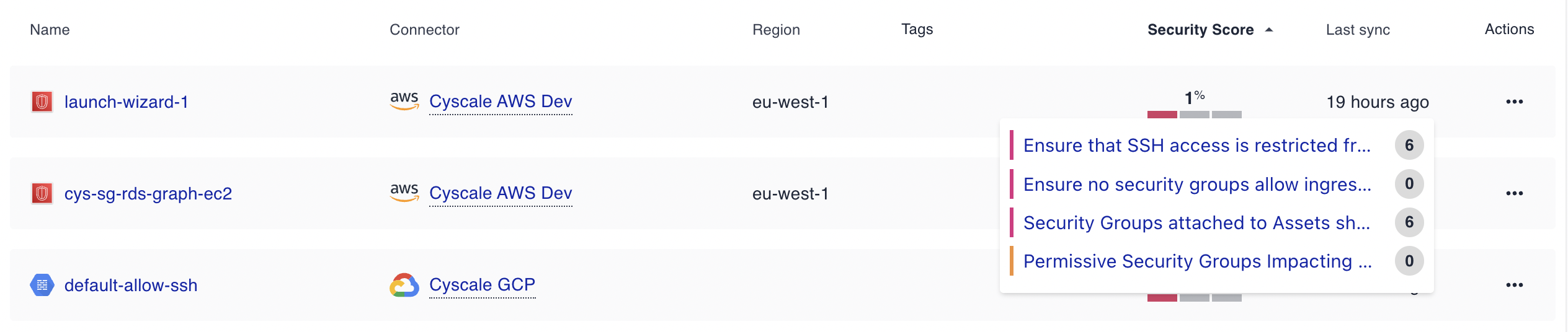# Asset Score

The asset score is a metric that indicates the security impact of an asset. It is calculated based on the controls that are relevant for that asset and any assets that are impacted by the failure. The asset score is a number between 0 and 100, where 0 means that the asset has a high impact on your security posture and 100 means the asset is compliant with all the controls that check it.

## How is the asset score calculated?​

The asset score is calculated based on the:

• number of controls the asset has failed
• the severity of the controls the asset has failed
• the number of assets that are impacted by the asset failing a particular control

For example, if an asset fails a control with high severity, the asset score will be lowered by 50%. If another control with high severity is failed by the same asset, the asset score will be lowered by another 50% from the new score, resulting in a score of 25%.If the asset also increases the risk of other assets, the score of the asset will be lowered for each of the impacted assets as if additional controls were failed by the asset.

So, if an asset fails a control with high severity and impacts 2 other assets, the asset score will be lowered recursively by 50%, resulting in a score of 13% (the numbers are rounded to the nearest integer).

You can see the number of impacted assets when you hover over the score. A number of 0 means that the asset does not impact any other assets except itself.For this example, we can see that the misconfigurations on this asset also impact 12 other assets, 6 for one control and 6 for another control.
Impacted assets are not guaranteed to be distinct, meaning that of the 12 impacted assets, some of them may be the same asset, but impacted by different controls.

## Severity factors​

As previously discussed, each control has a severity associated with it. The severity of a control is used to calculate the impact of the asset failing that control on the score. The severity of a control is one of the following:

• Low - the asset score is lowered by 10%
• Medium - the asset score is lowered by 25%
• High - the asset score is lowered by 50%

## How can I improve my asset score?​

You can improve the asset score by following the remediation steps for the controls that the asset has failed. The asset score will be recalculated after the next assessment.